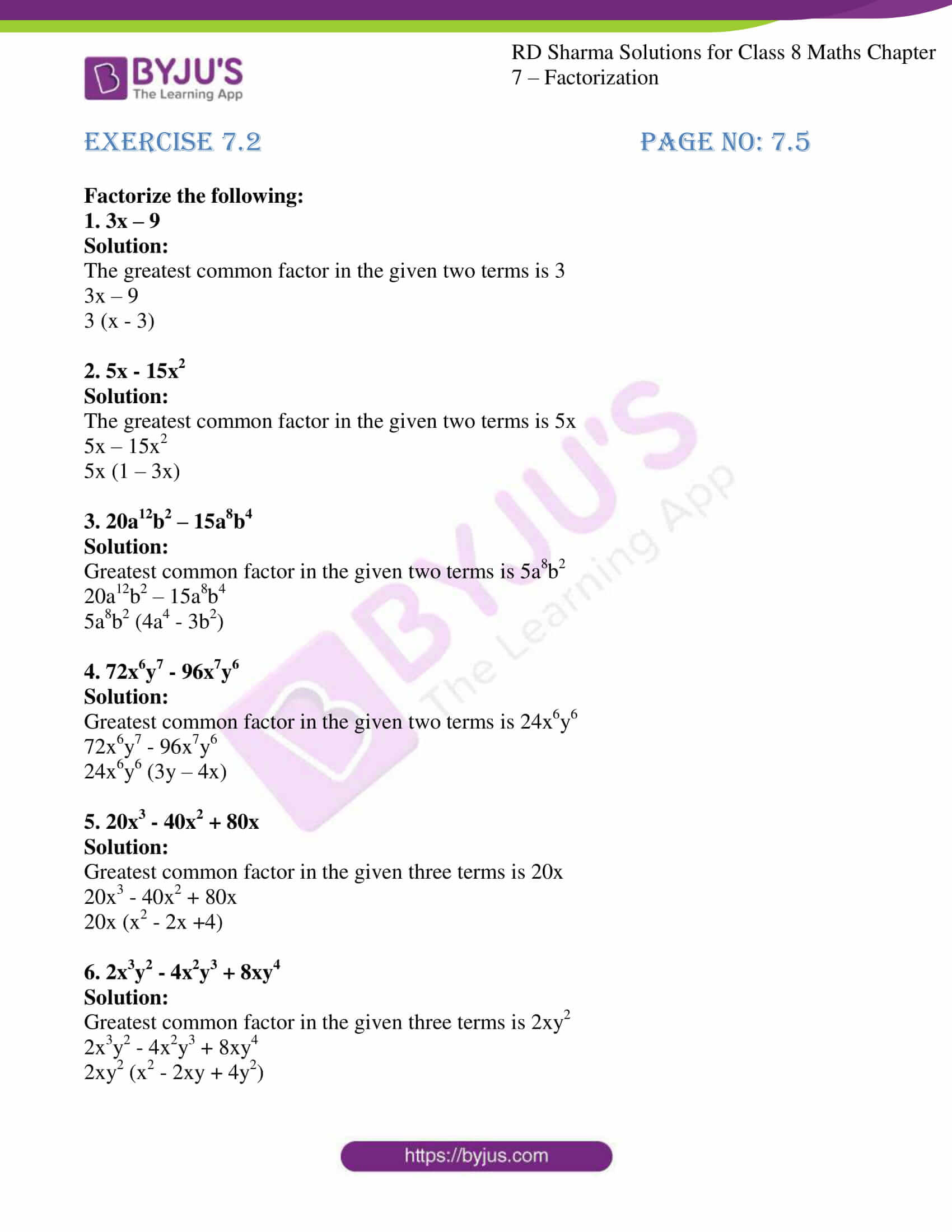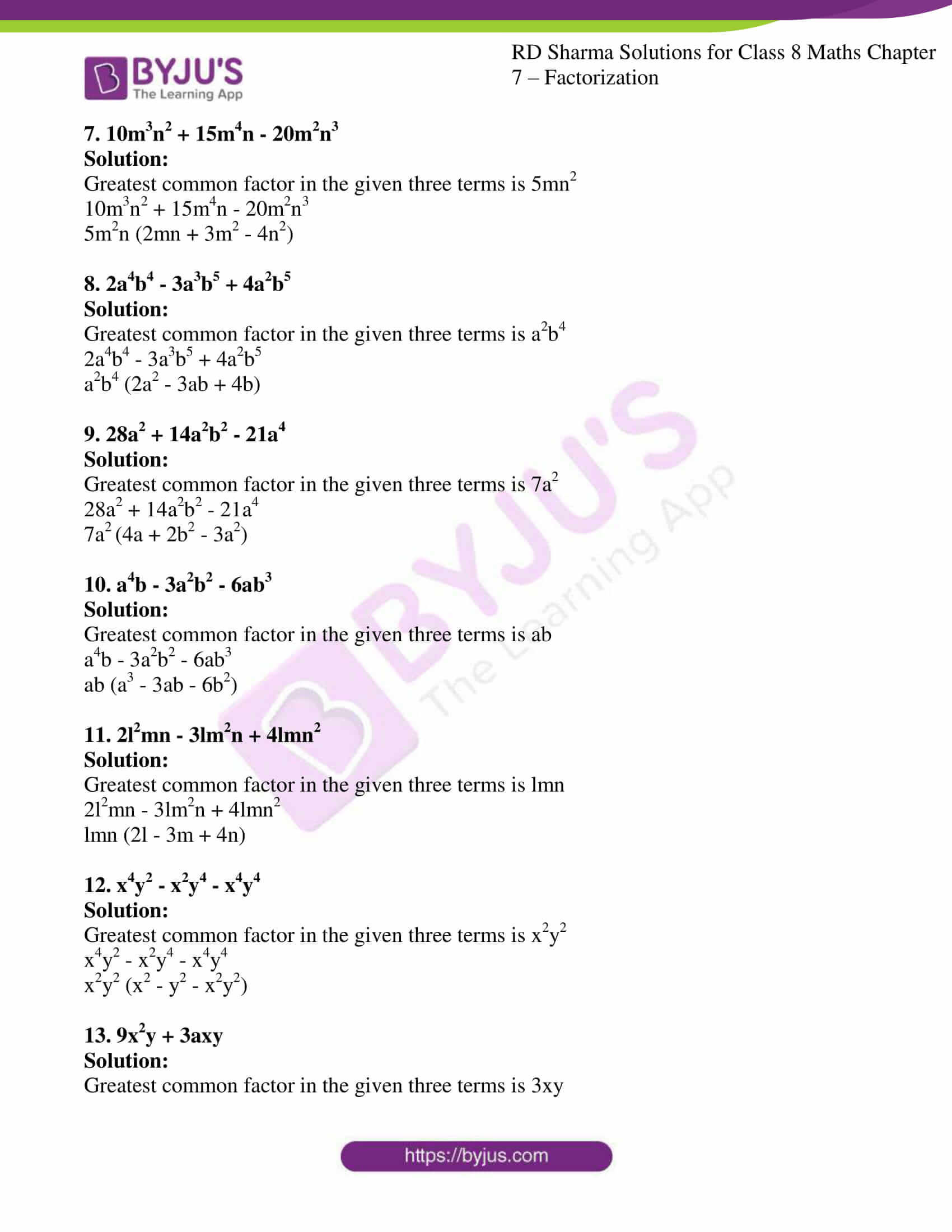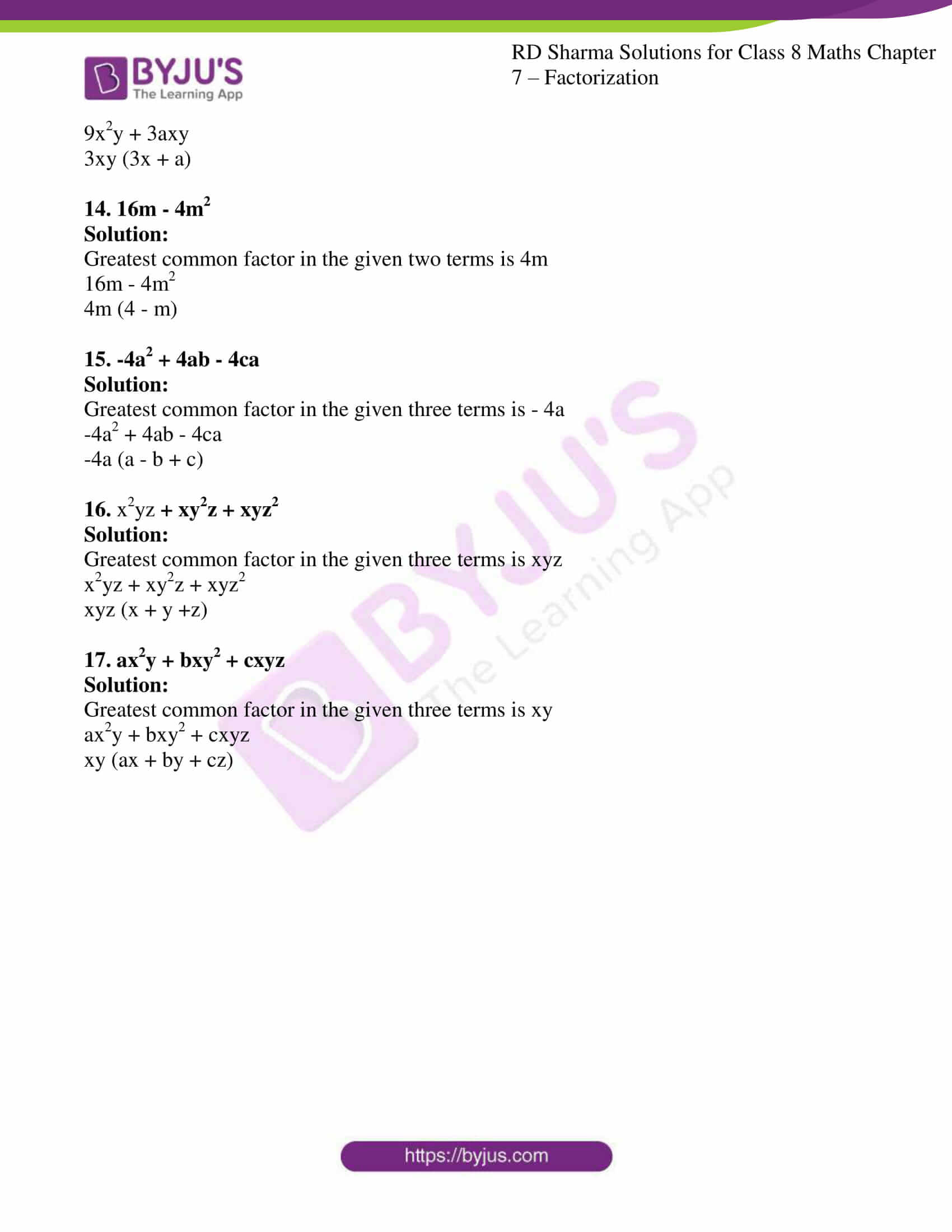# RD Sharma Solutions for Class 8 Maths Chapter - 7 Factorization Exercise 7.2

RD Sharma Solutions for Class 8 Maths Exercise 7.2 Chapter 7 Factorization are available here. The expert faculty at BYJU’S have formulated solutions based on the marks allotted for each step of a problem. The main objective is to help students in solving problems in turn which makes the subject easier to understand. Our experts have provided chapter-wise solutions which helps students perform well in the exam. Students can download RD Sharma Solutions from the links given below.

In Exercise 7.2 of Chapter 7 Factorization, we shall study the concepts based on the factorization of algebraic expressions when a common monomial factor occurs in each term.

## Download the pdf of RD Sharma Solutions For Class 8 Maths Exercise 7.2 Chapter 7 Factorization### Access Answers to RD Sharma Solutions for Class 8 Maths Exercise 7.2 Chapter 7 Factorization

#### EXERCISE 7.2 PAGE NO: 7.5

Factorize the following:

1. 3x – 9

Solution:

The greatest common factor in the given two terms is 3

3x – 9

3 (x – 3)

2. 5x – 15x2

Solution:

The greatest common factor in the given two terms is 5x

5x – 15x2

5x (1 – 3x)

3. 20a12b2 – 15a8b4

Solution:

Greatest common factor in the given two terms is 5a8b2

20a12b2 – 15a8b4

5a8b2 (4a4 – 3b2)

4. 72x6y7 – 96x7y6
Solution:

Greatest common factor in the given two terms is 24x6y6

72x6y7 – 96x7y6

24x6y6 (3y – 4x)

5. 20x3 – 40x2 + 80x

Solution:

Greatest common factor in the given three terms is 20x

20x3 – 40x2 + 80x

20x (x2 – 2x +4)

6. 2x3y2 – 4x2y3 + 8xy4

Solution:

Greatest common factor in the given three terms is 2xy2

2x3y2 – 4x2y3 + 8xy4

2xy2 (x2 – 2xy + 4y2)

7. 10m3n2 + 15m4n – 20m2n3

Solution:

Greatest common factor in the given three terms is 5mn2

10m3n2 + 15m4n – 20m2n3

5m2n (2mn + 3m2 – 4n2)

8. 2a4b4 – 3a3b5 + 4a2b5

Solution:

Greatest common factor in the given three terms is a2b4

2a4b4 – 3a3b5 + 4a2b5

a2b4 (2a2 – 3ab + 4b)

9. 28a2 + 14a2b2 – 21a4

Solution:

Greatest common factor in the given three terms is 7a2

28a2 + 14a2b2 – 21a4

7a2 (4a + 2b2 – 3a2)

10. a4b – 3a2b2 – 6ab3

Solution:

Greatest common factor in the given three terms is ab

a4b – 3a2b2 – 6ab3

ab (a3 – 3ab – 6b2)

11. 2l2mn – 3lm2n + 4lmn2

Solution:

Greatest common factor in the given three terms is lmn

2l2mn – 3lm2n + 4lmn2

lmn (2l – 3m + 4n)

12. x4y2 – x2y4 – x4y4

Solution:

Greatest common factor in the given three terms is x2y2

x4y2 – x2y4 – x4y4

x2y2 (x2 – y2 – x2y2)

13. 9x2y + 3axy

Solution:

Greatest common factor in the given three terms is 3xy

9x2y + 3axy

3xy (3x + a)

14. 16m – 4m2

Solution:

Greatest common factor in the given two terms is 4m

16m – 4m2

4m (4 – m)

15. -4a2 + 4ab – 4ca

Solution:

Greatest common factor in the given three terms is – 4a

-4a2 + 4ab – 4ca

-4a (a – b + c)

16. x2yz + xy2z + xyz2

Solution:

Greatest common factor in the given three terms is xyz

x2yz + xy2z + xyz2

xyz (x + y +z)

17. ax2y + bxy2 + cxyz

Solution:

Greatest common factor in the given three terms is xy

ax2y + bxy2 + cxyz

xy (ax + by + cz)